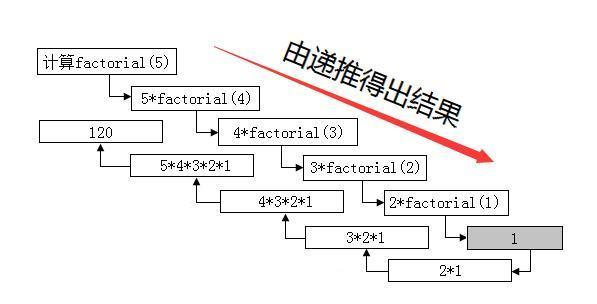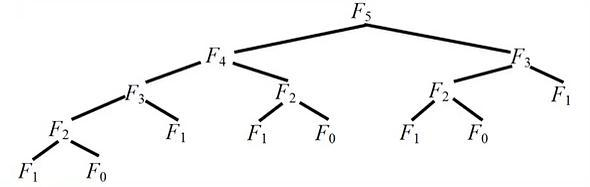## 经典算法｜递归和递归消除的迭代法

为什么80%的码农都做不了架构师？->>># 1 递归算法 Recursive Algorithm

if (条件)

return (不需要递归的简单答案);

else

return (递归调用同一函数)；

unsigned long factorial(unsigned long number)

{

if(number<=1);

reuturn 1;

else

return number*factorial(number-1);

}# 2 递归消除

2.1 利用栈结构消除递归

2.2 尾递归消除法

2.3 迭代法 Iterative Method

• 确定迭代变量；

• 建立迭代关系式

• 对迭代过程进行控制。

unsigned long factorial(unsigned long number)

{

unsigned long result = 1;

for(unsigned long i = number; i>=1; i--)

result *= i;

return result;

}

# 4 Fibonacci函数的递归和迭代实现

Fibonacci函数的递归实现

int f(int n)

{if (n==0) return 0;

elseif (n==1) return 1;.

else return (f(n-1)+f(n-2));

}Fibonacci函数的迭代实现

int f(int n)

{ int i, fn, fn_1 = 0, fn_2 = 1;

if (n <= 0) return n;

for ( i = 2; i<=n; ++i)

{ fn = fn_1 + fn_2;

fn_2 = fn_1; fn_1 = fn; }

return fn;

}